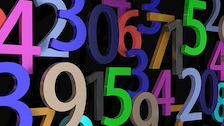# Playing with Mathematical SudokusWe are introducing a variation of the well-known Sudoku, by including mathematical constraints which challenge you to apply your knowledge of the four operations. For each grid size, there are four different puzzles, at each of three levels of difficulty, so plenty for you to get stuck into!

To introduce the Mathdokus, and to give a flavour of the kind of strategies that you may find useful, you may like to watch this video.

The last date for submitting solutions to the live problems is Monday 31 October.

### 3 by 3 Mathdokus

##### Age 7 to 14Challenge Level
Can you use the clues to complete these 3 by 3 Mathematical Sudokus?

### 4 by 4 Mathdokus

##### Age 7 to 14Challenge Level
Can you use the clues to complete these 4 by 4 Mathematical Sudokus?

### 5 by 5 Mathdokus

##### Age 7 to 16Challenge Level
Can you use the clues to complete these 5 by 5 Mathematical Sudokus?

### 6 by 6 Mathdokus

##### Age 7 to 16Challenge Level
Can you use the clues to complete these 6 by 6 Mathematical Sudokus?

### Mathdoku Editor

You can use the Mathdoku Editor to create your own Mathdokus.